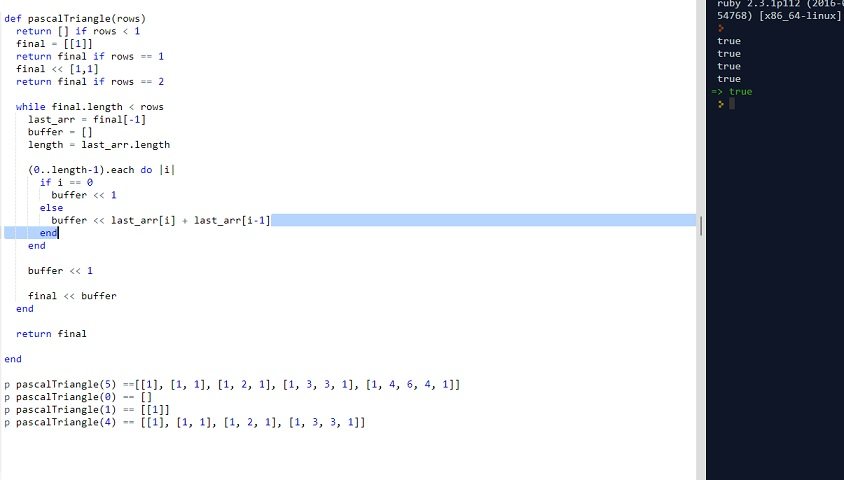# CLEAN CODE: PASCAL TRIANGLE | ARRAY

By: Saurav

2018-02-06 03:36:00 UTC

Given numRows, generate the first numRows of Pascal’s triangle.

Pascal’s triangle : To generate A[C] in row R, sum up A’[C] and A’[C-1] from previous row R - 1.

Example:

Given numRows = 5,

Return

[
,
[1,1],
[1,2,1],
[1,3,3,1],
[1,4,6,4,1]
]

InterviewBit

This is an observational question where you need to see the pattern. You need to write few test cases on the whiteboard and see the relationship between current and last row in the triangle.

Assumption: The row(sub-array) are 0 indexed

Algo:
The first element of current will be 1
The second to last_row_length-1 for current row will be equal to last_row[index] + last_row[index-1]
The last element will be 1

Lets write a few test cases and see it in action:

```p pascalTriangle(5) ==[, [1, 1], [1, 2, 1], [1, 3, 3, 1], [1, 4, 6, 4, 1]]
p pascalTriangle(0) == []
p pascalTriangle(1) == []
p pascalTriangle(4) == [, [1, 1], [1, 2, 1], [1, 3, 3, 1]]```

```def pascalTriangle(rows)
return [] if rows < 1
final = []
return final if rows == 1
final << [1,1]
return final if rows == 2

while final.length < rows
last_arr = final[-1]
buffer = []
length = last_arr.length

(0..length-1).each do |i|
if i == 0
buffer << 1
else
buffer << last_arr[i] + last_arr[i-1]
end
end

buffer << 1

final << buffer
end

return final

end ```As we see all our tests pass and the code looks good as well.

Looks good to me.

### Let me know what you think! twitter: sprakash24octlinkedin

Owned & Maintained by Saurav Prakash

If you like what you see, you can help me cover server costs or buy me a cup of coffee though donation :)